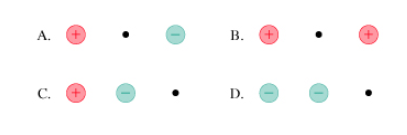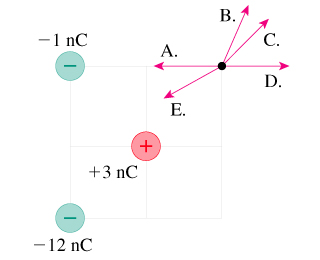# Problem: 1. In which case does the electric field at the dot have the largest magnitude? A, B, C, or D2. Three point charges are arranged as shown in the figure below.Which arrow best represents the direction of the electric field vector at the position of the dot? A, B, C, D, or E

🤓 Based on our data, we think this question is relevant for Professor Jyotishmati's class at COLLIN.

###### FREE Expert Solution

1.

Electric field:

$\overline{){\mathbf{E}}{\mathbf{=}}\frac{\mathbf{k}\mathbf{q}}{{\mathbf{r}}^{\mathbf{2}}}}$

The electric field is proportional to q and inversely proportional to r.###### Problem Details

1. In which case does the electric field at the dot have the largest magnitude? A, B, C, or D2. Three point charges are arranged as shown in the figure below.Which arrow best represents the direction of the electric field vector at the position of the dot? A, B, C, D, or E